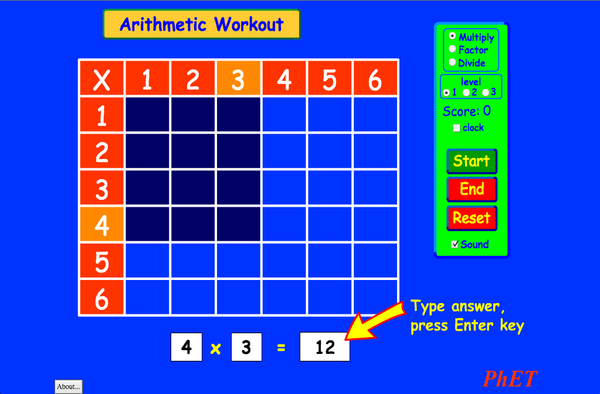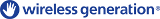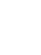# ArithmeticDownloadEmbed close Embed a running copy of this simulation Use this HTML to embed a running copy of this simulation. You can change the width and height of the embedded simulation by changing the "width" and "height" attributes in the HTML. Embed an image that will launch the simulation when clicked
Click to Run
Use this HTML code to display a screenshot with the words "Click to Run". Multiplication PhET is supported byand educators like you.Back to HTML5 Version

### Topics

• Multiplication

### Description

Remember your multiplication tables? Practice your multiplication, division, and factoring skills with this exciting game. No calculators allowed!

### Sample Learning Goals

• Explain how multiplication tables help understand multiplication, factoring, and division.
• Use an array model to understand multiplication, factoring, and division.
• Increase accuracy in multiplying, factoring and dividing.
• Develop multiple strategies for arithmetic problems.

### Standards Alignment

#### Common Core - Math

3.OA.A.1
Interpret products of whole numbers, e.g., interpret 5 × 7 as the total number of objects in 5 groups of 7 objects each. For example, describe a context in which a total number of objects can be expressed as 5 × 7.
3.OA.A.2
Interpret whole-number quotients of whole numbers, e.g., interpret 56 ÷ 8 as the number of objects in each share when 56 objects are partitioned equally into 8 shares, or as a number of shares when 56 objects are partitioned into equal shares of 8 objects each. For example, describe a context in which a number of shares or a number of groups can be expressed as 56 ÷ 8.
3.OA.A.4
Determine the unknown whole number in a multiplication or division equation relating three whole numbers. For example, determine the unknown number that makes the equation true in each of the equations 8 × ? = 48, 5 = _ ÷ 3, 6 × 6 = ?
3.OA.B.5
Apply properties of operations as strategies to multiply and divide.2 Examples: If 6 × 4 = 24 is known, then 4 × 6 = 24 is also known. (Commutative property of multiplication.) 3 × 5 × 2 can be found by 3 × 5 = 15, then 15 × 2 = 30, or by 5 × 2 = 10, then 3 × 10 = 30. (Associative property of multiplication.) Knowing that 8 × 5 = 40 and 8 × 2 = 16, one can find 8 × 7 as 8 × (5 + 2) = (8 × 5) + (8 × 2) = 40 + 16 = 56. (Distributive property.)
3.OA.B.6
Understand division as an unknown-factor problem. For example, find 32 ÷ 8 by finding the number that makes 32 when multiplied by 8.
3.OA.C.7
Fluently multiply and divide within 100, using strategies such as the relationship between multiplication and division (e.g., knowing that 8 × 5 = 40, one knows 40 ÷ 5 = 8) or properties of operations. By the end of Grade 3, know from memory all products of two one-digit numbers.
3.OA.D.9
Identify arithmetic patterns (including patterns in the addition table or multiplication table), and explain them using properties of operations. For example, observe that 4 times a number is always even, and explain why 4 times a number can be decomposed into two equal addends.
Version 2.03

### Teacher TipsOverview of sim controls, model simplifications, and insights into student thinking ( PDF ).

### Teacher-Submitted Activities

How do PhET simulations fit in my middle school program?Sarah Borenstein MS Other Biology
Earth Science
Chemistry
Physics
MS and HS TEK to Sim Alignment Elyse Zimmer HS
MS
Other Chemistry
Physics
Biology
Math facts - Using the Multiplication Chart Tapan Sarkar MS Lab Mathematics
Back to the Basics Nyetta Abernathy K-5
MS
HS
HW
Lab
Mathematics
Discovering divisibility rules Stacy Larson HS
MS
Lab Mathematics
Juego de Aritmética (Basado en Indagación)Trish Loeblein, traducido por Diana López HS
MS
Guided
HW
Mathematics
곱셈 나눗셈 연습 SIM 사용설명서 이화국(Wha Kuk Lee) MS
K-5
CQs
HW
Demo
Physics
Mathematics
ARITHMETIC_DIVISON Arlete Cabral K-5 Lab Mathematics
OBJETO DE APRENDIZAGEM: ARITHMETIC Arlete Moura de Oliveira Cabral e Maria Cleide da Silva Barroso K-5 Guided Mathematics
Operações Aritméticas Fundamentais Georgyana Cidrão; Francisco Régis Vieira Alves K-5 Lab
Guided
Mathematics
Language Downloador RunTips
Afrikaans All AfrikaansRekenkunde
Albanian All shqipAritmetika
Amharic All Amharicየሒሳብ ስሌቶች
Arabic All العربيةarithmetic
Arabic (Saudi Arabia) All العربية (السعودية)arithmetic
Basque All EuskaraAritmetika
Bosnian All BosanskiARITMETIKA
Chinese (Simplified) All 中文 (中国)算术
Chinese (Traditional) All 中文 (台灣)算數
Croatian All hrvatskiAritmetika
Danish All DanskAritmetisk
Dutch All NederlandsRekenen
Estonian All EestiAritmeetika
French All françaisArithmétique
Georgian All ქართულიარითმეტიკა
German All DeutschRechnen
Greek All ΕλληνικάΑριθμητικός
Hebrew All עבריתחשבון
Hungarian All magyarSzorzótábla játék
Indonesian All Bahasa IndonesiaAritmatika
Italian All italianotabelline
Japanese All 日本語計算
Kazakh All KazakhАрифметика
Korean All 한국어곱셈 나눗셈 연습
Latvian All LatviešuAritmētika
Macedonian All македонскиАРИТМЕТИКА
Marathi All मराठीअंकगणित
Persian All فارسیحساب
Polish All polskiArytmetyka
Portuguese (Brazil) All português (Brasil)Aritmética
Romanian All românăAritmetica
Russian All русскийАрифметика
Serbian All СрпскиАРИТМЕТИКА
Slovak All SlovenčinaAritmetika
Spanish All españolAritmética
Spanish (Mexico) All español (México)Aritmética
Spanish (Peru) All español (Perú)Aritmética
Swahili All SwahiliHesabu
Swedish All svenskaMatteräkning
Turkish All TürkçeAritmetik
Turkmen All TurkmenArifmetika
Vietnamese All Tiếng ViệtSố học
Windows Macintosh Linux
Microsoft Windows
XP/Vista/7/8.1/10
Macromedia Flash 9 or later
OS X 10.9.5 or later
Macromedia Flash 9 or later
Macromedia Flash 9 or later
Design Team Third-party Libraries Thanks To